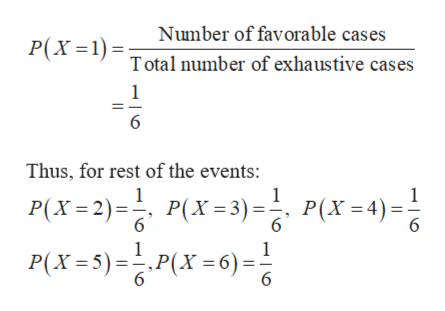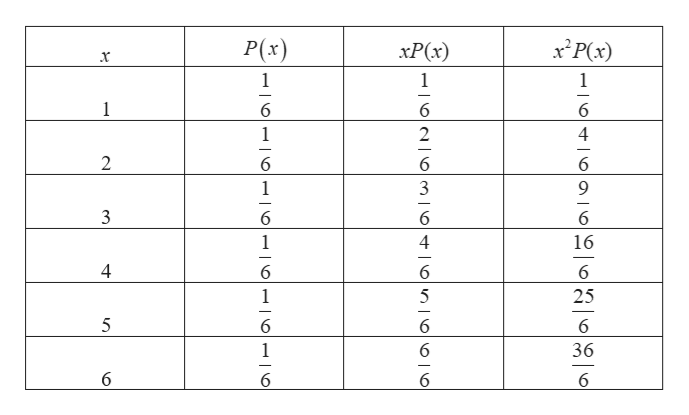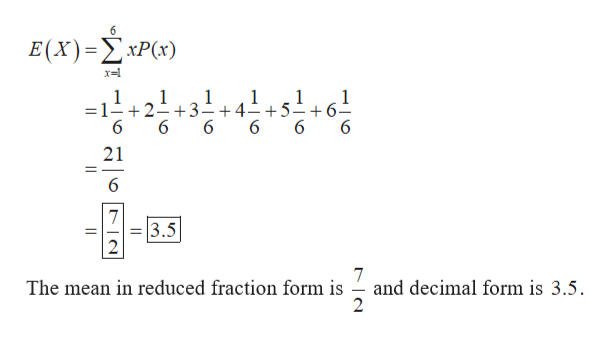MAC 15First, consider an 'experiment' where we will toss a fair die and let  X = the number of dots facing up. of course the possible values for X are 1, 2, 3, 4, 5 and 6 and each of these is equally likely. Show the probability distribution for X using the first two columns in the table below. Avoid rounding errors, show the probabilities as fractions - not as decimals. Next fill in the next two columns using fractions. Don't reduce fractions as you'll want to have a common denominator in each column. * means multiplyx P(X = x)x * P(X = x)x^2 * P(X = x)1   2   3   4   5   6   SUM    a) Express the Expected Value of X, also known as the mean, u, as both a reduced fraction and as a decimal b) show your work to find variance and standard deviation in both fraction and decimal.

Question

MAC 15

First, consider an 'experiment' where we will toss a fair die and let  X = the number of dots facing up. of course the possible values for X are 1, 2, 3, 4, 5 and 6 and each of these is equally likely. Show the probability distribution for X using the first two columns in the table below. Avoid rounding errors, show the probabilities as fractions - not as decimals. Next fill in the next two columns using fractions. Don't reduce fractions as you'll want to have a common denominator in each column.

* means multiply

 x P(X = x) x * P(X = x) x^2 * P(X = x) 1 2 3 4 5 6 SUM

a) Express the Expected Value of X, also known as the mean, u, as both a reduced fraction and as a decimal

b) show your work to find variance and standard deviation in both fraction and decimal.

Step 1

Consider the random variable X, that is defined as the number of dots facing up in a fair die. The values of X are 1,2,3,4,5 and 6, which are equally likely.

The probability of getting X is equal to 1:help_outlineImage TranscriptioncloseNumber of favorable cases P(X Total number of exhaustive cases 1 6 Thus, for rest of the events: 1 1 P(X2)P3)P(X=4)= P(X= 5) =승,P(X =6) = P(X 3) P(X4) 6 6 6 1 fullscreen
Step 2

The completed table is shown below:help_outlineImage TranscriptionclosexPx) P(x) xP(x) 1 2 3 1 4 6 -|0N6mo 0n|0|0| 0 fullscreen
Step 3

a)

The expected value of X can b...help_outlineImage Transcriptionclose6 E(X) -ΣxP(x) 1 =1- +2- +3-+4- +5 6 1 1 1 1 6 6 1 6 6 6 21 _ 6 7 3.5 7 and decimal form is 3.5 The mean in reduced fraction form is fullscreen

Want to see the full answer?

See Solution

Want to see this answer and more?

Our solutions are written by experts, many with advanced degrees, and available 24/7

See Solution
Tagged in

Other